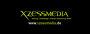[Solved] Simple Actor example Author Message
xzessmediaMemberPost: #1 [Solved] Simple Actor example Hi there, can anybody explain me in short, how Actors work? I studied all Tutorials about Actors and Physical Objects but the important topic for me is, Actors combined with meshes which seem to not be handled in the tutorials... I also read the wiki page about Actors and watched some videos from scarlet. The following Draw function should sync the mesh with the actors matrix but it is always out of sync... so if i apply physics, only the actor moves... any help is really appreciated... Code: ```void Ship::create(Game.ObjParams &obj) {       scale          =  obj.scale3();       mesh           =  obj.mesh();       material       =  obj.material();       phys           =  obj.phys();              _matrix        =  obj.matrixFinal().normalize();       _matrixscaled  =  _matrix;       _matrixscaled.scaleOrn(scale);              angle          = Angle(obj.matrixFinal().z.xz())-PI_2;       speed          = 5;              if(phys)       {          actor.create(*phys, 1, scale).matrix(_matrix);          actor.obj(this);          actor.group(AG_CONTROLLER);       } } ... ... ... uint Ship::drawPrepare() {    if(mesh && Frustum(mesh->box, _matrix))    {       MaterialLock = material();       mesh->draw(_matrixscaled);              MaterialLock = NULL;    }    return NULL; }``` Thank you very very much for all answers!! I guess your answers will help many people to understand how it works! ok sorry for doublepost but i solved it myself... the solution is very simple mesh->draw(actor.matrix().normalize()); just used the wrong matrix (This post was last modified: 06-12-2013 10:08 PM by xzessmedia.)
06-12-2013 09:50 PM
Esenthel